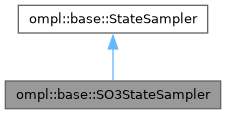ompl::base::SO3StateSampler Class Reference

State space sampler for SO(3), using quaternion representation
More...

#include <ompl/base/spaces/SO3StateSpace.h>

Inheritance diagram for ompl::base::SO3StateSampler:[legend]

## Public Member Functions

SO3StateSampler (const StateSpace *space)
Constructor.

void sampleUniform (State *state) override
Sample a state.

void sampleUniformNear (State *state, const State *near, double distance) override
To sample unit quaternions uniformly within some given distance, we sample a 3-vector from the R^3 tangent space. This vector is drawn uniformly random from a 3D ball centered at the origin with radius distance. The vector is then "wrapped" around S^3 to obtain a unit quaternion uniformly distributed around the identity quaternion within given distance. We pre-multiply this quaternion with the quaternion near to center the distribution around near.

void sampleGaussian (State *state, const State *mean, double stdDev) override
Sample a state such that the expected distance between mean and state is stdDev. More...Public Member Functions inherited from ompl::base::StateSampler
StateSampler (const StateSampler &)=delete

StateSampleroperator= (const StateSampler &)=delete

StateSampler (const StateSpace *space)
Constructor.Protected Attributes inherited from ompl::base::StateSampler
const StateSpacespace_
The state space this sampler samples.

RNG rng_
An instance of a random number generator.

## Detailed Description

State space sampler for SO(3), using quaternion representation

Definition at line 47 of file SO3StateSpace.h.

## ◆ sampleGaussian()

 void ompl::base::SO3StateSampler::sampleGaussian ( State * state, const State * mean, double stdDev )
overridevirtual

Sample a state such that the expected distance between mean and state is stdDev.

To sample a unit quaternion from a Gaussian distribution, we sample a 3-vector from the R^3 tangent space using a 3D Gaussian with zero mean and covariance matrix equal to diag(stdDev^2, stdDev^2, stdDev^2). This vector is "wrapped" around S^3 to obtain a Gaussian quaternion with zero mean. We pre-multiply this quaternion with the quaternion mean to get the desired mean.

Implements ompl::base::StateSampler.

Definition at line 119 of file SO3StateSpace.cpp.

The documentation for this class was generated from the following files: